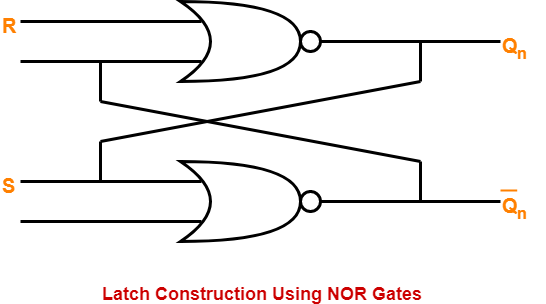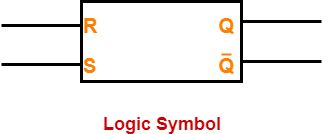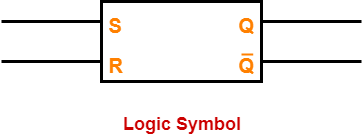## Latch-

A latch may be defined as-

 A latch is basically an unclocked flip flop. OR A latch is the basic building block using which clocked flip flops are constructed.

## Latch Construction-

There are following two methods for constructing a latch-1. By using 2 NOR gates
2. By using 2 NAND gates

### Logic Circuit-

The logic circuit for a latch constructed using NOR gates is as shown below-While constructing a latch using NOR gates, it is compulsory to consider-

• Reset input R in normal output Qn.
• Set input S in complemented output Q’n.

### Logic Symbol-

The logic symbol for a latch constructed using NOR gates is as shown below-### Truth Table-

The truth table for a latch constructed using NOR gates is as shown below-

 INPUTS OUTPUTS R S Qn (Present State) Qn+1 (Next State) 0 0 0 0 0 0 1 1 0 1 0 1 0 1 1 1 1 0 0 0 1 0 1 0 1 1 0 Indeterminate 1 1 1 Indeterminate

### Truth Table

The above truth table may be reduced as-

 INPUTS OUTPUTS REMARKS R S Qn (Present State) Qn+1 (Next State) States and Conditions 0 0 X Qn Hold state condition R = S = 0 0 1 X 1 Set state condition R = 0 , S = 1 1 0 X 0 Reset state condition R = 1 , S = 0 1 1 X Indeterminate Indeterminate state condition R = S = 1

### Logic Circuit-

The logic circuit for a latch constructed using NAND gates is as shown below-While constructing a latch using NAND gates, it is compulsory to consider-

• Set input S in normal output Qn.
• Reset input R in complemented output Q’n.

### Logic Symbol-

The logic symbol for a latch constructed using NAND gates is as shown below-### Truth Table-

The truth table for a latch constructed using NAND gates is as shown below-

 INPUTS OUTPUTS S R Qn (Present State) Qn+1 (Next State) 0 0 0 Indeterminate 0 0 1 Indeterminate 0 1 0 1 0 1 1 1 1 0 0 0 1 0 1 0 1 1 0 0 1 1 1 1

### Truth Table

The above truth table may be reduced as-

 INPUTS OUTPUTS REMARKS S R Qn (Present State) Qn+1 (Next State) States and Conditions 0 0 X Indeterminate Indeterminate state condition S = R = 0 0 1 X 1 Set state condition S = 0 , R = 1 1 0 X 0 Reset state condition S = 1 , R = 0 1 1 X Qn Hold State condition S = R = 1

### Truth Table

To gain better understanding about Latch in Digital Electronics,

Watch this Video Lecture

Next Article- Types of Flip-Flops

Get more notes and other study material of Digital Design.

Watch video lectures by visiting our YouTube channel LearnVidFun.